## 12.1 Adapting the general algorithm

In the last chapter we presented the following general algorithm for working with active charts and agendas, and claimed that by making small changes to this algorithm we would be able to make it work either bottom-up or top-down:

1. Make initial chart and agenda.

2. Repeat until agenda is empty:

1. Take first arc from agenda.

2. Add arc to chart. (Only do this if edge is not already on the chart!)

3. Use the fundametal rule to combine this arc with arcs from the chart. Any edges obtained in this way should be added to the agenda.

4. Make hypotheses (i.e., active edges) about new constituents based on the arc and the rules of the grammar. Add these new arcs to the agenda.

End repeat

3. See if the chart contains a passive edge from the first node to the last node that has the label s. If yes'', succeed. If no'', fail.

In the last chapter we showed how to make this work bottom-up. Now we'll see how to make it work top-down.

There are two main changes we have to make. First, and most fundamentally, we have to think about the ways rules are used to make active edges. That is, we need to rethink step 2d of the algorithm. In addition, we need to make the initial chart and agenda differently. Let's discuss each issue in turn.

### 12.1.1 Top-down rule selection

Let's first look at how to change 2d. When working bottom-up, we were interested in using rules right-to-left. We would start with structure that we knew we had (for example, perhaps we have a passive edge that tells us that there is a PN between positions 0 and 1) and then find a rule that we could use right-to-left to make use of this fact (for example, reading the rule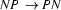right to left we could deduce that we had an NP between positions 0 and 1). Summing up: working bottom up, we read the rules right-to-left, and start with the information in passive edges.

However, when we work top-down, we do precisely the opposite: we read the rules left-to-right and start with the information in active edges. Let's see why.

Suppose we have an active edge that starts at position 0 and is trying to build an S out of an NP and a VP in that order, and suppose it has found neither the NP nor the VP (so the arc label would be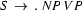. Further, suppose we can't apply the fundamental rule (that is, there is no passive edge that tells us that there is an NP starting at position 0). What should we do? Well, think about it. Since we are hypothesizing that it is possible to build an S out of an NP and a VP in that order starting at position 0, we should try and find grammar rules that will let us do this. In particular, we should look for grammar rules that have NP on the left-hand-side, for these are the rules that tell us how to build NPs --- if we want that sentence, we need an NP! We then use these rules to add a new active edge at position 0. For example, if we have the rule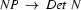, we should add an active edge at position 0 that is trying to build an NP out of a Det and an N in that order.

In short: working top-down, we read the rules left-to-right, and start with the information in active edges. So in the top-down case we should change step 2d to read: If the edge you added was active, try to select a rules that can be used with the edge you have just added to make new active edges. Add these new edges to the agenda.'' This is the main point we need to make about rule selection, but there is another, less important issue, due to which we will make slight changes to the way we represent our grammar.

### 12.1.2 Initializing Chart and Agenda

In the bottom-up algorithm, we form an initial agenda containing passive arcs that represent all possible categories of all the words in the input string. Now, this is clearly important information, and it's information we will continue to need when working top-down --- but since in the top-down case new rules can only be predicted from active edges, we won't be able to use the passive arcs representing categories of words in the beginning. Only when we have worked our way down to the pre-terminal nodes will this information be important. We therefore write those arcs directly into the chart. What we do need though is at least one active edge on the agenda to start the parsing process.

So what active edge(s) should we start with? Recall that active edges can be thought of as hypotheses about structure --- and there is one very obvious hypothesis to make about the structure of the input string, namely, that it is a sentence.

This suggests that, right at the start of the top-down algorithm, we should look at our grammar and find all the rules that let us make sentences. We should then use these rules to make active edges. Where should these active edges start from? At position 0. After all, we want to show that we have an S that starts at position 0 and spans the entire sentence.

For example, if we have the rule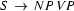in our grammar, we should add at position 0 an active edge that wants to make an S out of an NP and a VP in that order, at position 0. And if we have coordination rules, for example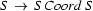, where coord can be and'', or'', but'', and so on, then we should also add an active edge at position 0 that is trying to build an S out of an S followed by a coordination word followed by another S.

The initial state of chart and agenda for the sentence Mia danced and the grammar of the last chapter would look like this: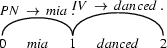1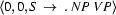2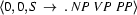Patrick Blackburn and Kristina Striegnitz
Version 1.2.4 (20020829)